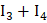# In the following questions, a series of numbers is given which follow certain rules.  One of the number is missing.  Choose the missing number from the alternatives given below. 9, 12, 11, 14, 13, ?, 15 (a) 17 (b) 16 (c) 19 (d) 21

## Question ID - 100688 :- In the following questions, a series of numbers is given which follow certain rules.  One of the number is missing.  Choose the missing number from the alternatives given below. 9, 12, 11, 14, 13, ?, 15 (a) 17 (b) 16 (c) 19 (d) 21

3537

16

Next Question :

The moments of inertia of a thin square plateof uniform thickness about an axis passing through the centreand perpendicular to the plate area)b)c)d)(whereandare, respectively, the moments of inertia about axes 1, 2, 3 and 4, where axes are in the plane of the plate)